Liquidity Index Of Soil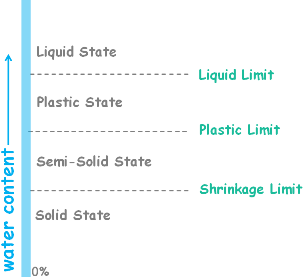Depending upon the water content soil may appear in one of these four states.

Liquid State

Plastic State

Semi Solid State and

Solid State

Water content at which the soil changes from one of these states to another are called consistency limits. These limits are:

Liquid Limit

Plastic Limit

Shrinkage Limit

In liquid state water content of the soil is such that it behaves like liquid and offers no resistance to flow.

When water content of this soil drops it gains shear strength and develops resistance to flow. After reaching certain water content named as liquid limit soil enters into a state called plastic state of soil.

In plastic state soil can be moulded in any shape due to a property called plasticity.

When water content of the soil is further reduced, after reaching another certain water content named as Plastic limit, soil enters into another state called semi solid state.

A quantity liquidity index is defined to understand the consistency of soil and it is represented by IL.

It is defined as the ratio of the difference between the natural water content of the soil and its plastic limit to its plasticity index. We write it mathematically as this.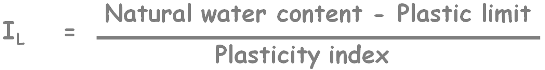We know plasticity index of a soil is difference between its liquid limit and plastic limit. So expression becomes this.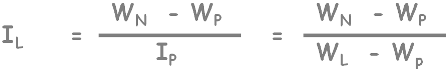We can determine the natural water content WN of the soil by any of the water content determination method.

When soil’s natural water content is equal to its plastic limit then the value of the liquidity index is zero.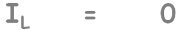Now if soil’s natural water content increases to its liquid limit then numerator and denominator will be equal and liquidity index value increases from zero to 1.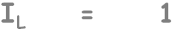If we further increase the natural water content of soil liquidity index increases past 1 as only numerator will increase but denominator will remain constant.We can notice if a soil is in plastic state then its liquidity index varies from 0 to 1.

From these observations we can see liquidity index of soil begins from zero at plastic limit and with increase in the water content soil’s liquidity index increases and becomes 1 at liquid limit. Now water content is further increased the value of liquidity index becomes greater than 1 and that indicates soil is in liquid state and behaves like liquid. At water content lower than plastic limit, soil is relatively harder and brittle in nature. Here liquidity index of the soil will be negative.

In general we can say with the increase in water content of the soil its liquidity Index increases and with it soil’s liquidity increases and firmness decreases.

We must note here that Liquidity index is used for the same purpose as the consistency index that is to scale the water content of the soil. Consistency index and liquidity index are related to each other as sum of Liquidity index and consistency index will always be equal to 1.We can see it by adding both of them.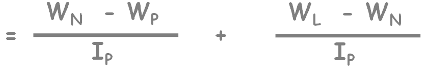Here represent plasticity index as WL minus Wp and add the equation.WN and WN will get cancelled and we will have this. Both are equal so here we get the 1.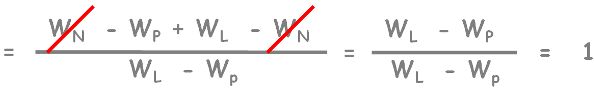But we only have to remember this.So in this relationship we can see at plastic limit consistency index value is 1 so liquidity index value is zero and at liquid limit consistency index value is zero so liquidity index value is 1.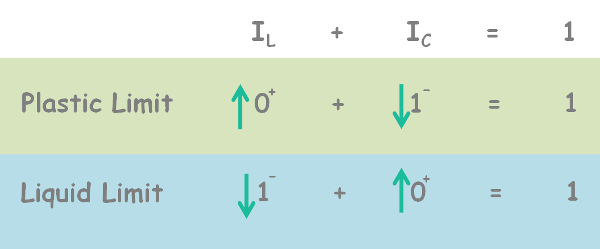Also if any of these quantities increases or decreases then the other quantity will decrease or increase in the same amount so as to maintain the sum equal to 1.

Tags : Liquidity Index

Published on :2019-11-20